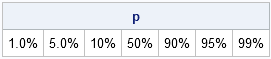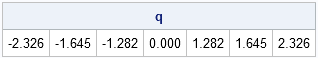"How do I apply a format to a vector of values in IML? In the DATA step, I can just call the PUTN function.”

This question came from a SAS customer that I met recently at a conference. My reply? Use the PUTN function, but send it a vector of values.

In particular, there is no need to write a loop. Just put in a vector of numbers and you’ll get out a character vector that contains the formatted values:

```proc iml; v = {0.01 0.05 0.1 0.5 0.9 0.95 0.99}; p = putn(v, "Percent6.3"); print p;```You can send a SAS/IML matrix to almost every DATA step function. For example, to compute the quantiles of the standard normal distribution that correspond to the values of v, just call the QUANTILE function in Base SAS:

```q = quantile("Normal", v); print q[format=6.3];```Neither the PUTN function nor the QUANTILE function are explicitly listed in the Language Reference section of the SAS/IML User’s Guide, because they are not technically part of the SAS/IML language. However, they are mentioned in the section titled "Base SAS Functions Accessible from SAS/IML Software."

ShareDistinguished Researcher in Computational Statistics

Rick Wicklin, PhD, is a distinguished researcher in computational statistics at SAS and is a principal developer of SAS/IML software. His areas of expertise include computational statistics, simulation, statistical graphics, and modern methods in statistical data analysis. Rick is author of the books Statistical Programming with SAS/IML Software and Simulating Data with SAS.

1.If I want use Lag function in Base/SAS, how do I do?

2.LAG is a function that IML does not support in SAS 9.2 (but will in the next release). You can write a simple module to compute the lag of a column vector:

proc iml;
start lag(x, d);
n = nrow(x);
y = j(n, 1, .);
if n >= d then
y[d+1:n] = x[1:n-d];
return( y );
finish;

x = {1,2,3,4,5};
x1 = lag(x, 1);
x2 = lag(x, 2);
print x1 x2;

3.Rick Wicklin on